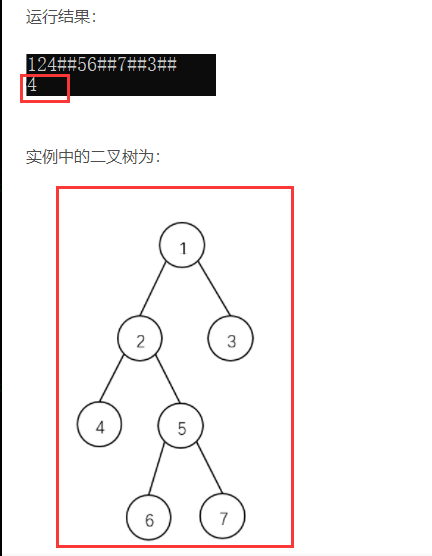2021-06-23 19:40

# 用二叉链表创建一棵二叉树并利用递归算法求它的高度

• 点赞
• 写回答
• 关注问题
• 收藏
• 邀请回答

#### 1条回答默认 最新

•CSDN专家-黄老师 2021-06-23 19:49
已采纳
``````#include<bits/stdc++.h>
using namespace std;

typedef struct BNode{
char data;
struct BNode *lchild;
struct BNode *rchild;
}BNode;

const int N = 100;
char str[N]; //存储先序遍历序列
int i; //标记处理到哪一个字符了
BNode *BulidTree(){
if(str[i] == '#'){
i++; //处理下一个字符
return NULL;
}else{
//新建一个结点
BNode *p = (BNode *)malloc(sizeof(BNode));
p->data = str[i];
p->lchild = NULL;
p->rchild = NULL;
i++;
p->lchild = BulidTree();
p->rchild = BulidTree();
return p;
}
}

//非递归算法
int Depth(BNode *root){
int level = 0; //level为层数
BNode *last = root;//last为下一层的最右结点
if(root == NULL){ //树空，则高度为0
return 0;
}
queue<BNode *> treenode; //申请一个队列
treenode.push(root); //根结点入队
while(!treenode.empty()){ //队不空时循环
BNode *p = treenode.front(); //队首
treenode.pop(); //根结点出队
if(p->lchild != NULL){ //如果存在左子树，则左子树根结点入队
treenode.push(p->lchild);
}
if(p->rchild != NULL){ //如果存在右子树，则右子树根结点入队
treenode.push(p->rchild);
}
if(p == last){ //如果刚才出队的是该层最右结点
level++; //层数加1
last = treenode.back(); //last指向下层
}
}
return level;
}

//递归算法
// int Depth2(BNode *root){
//     if(root == NULL){ //空树，高度为0
//         return 0;
//     }
//     int left = Depth2(root->lchild); //左子树高度
//     int right = Depth2(root->rchild); //右子树高度
//     return (left>right? left+1 : right+1); //树的高度为最大子树的高度加上根结点
// }

int main(){
scanf("%s",str);
i = 0;
BNode *root = BulidTree();
int level = Depth(root);
printf("%d\n",level);
return 0;
}
``````如果对你有帮助，可以点击我这个回答右上方的【采纳】按钮，给我个采纳吗，谢谢

点赞 评论